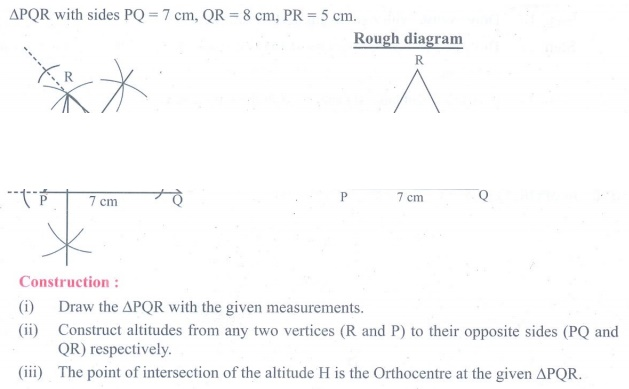Home | | Maths 9th std | Exercise 4.5: Construction of the Centroid and Orthocentre of Triangle

# Exercise 4.5: Construction of the Centroid and Orthocentre of Triangle

Maths : Practical Geometry : Book Back, Exercise, Example Numerical Question with Answers, Solution: Exercise 4.5: Construction of the Centroid and Orthocentre of Triangle

Construction of the Centroid of a Triangle and Orthocentre of a Triangle

Exercise 4.5

1. Construct the ΔLMN such that LM=7.5cm, MN=5cm and LN=8cm. Locate its centroid.2. Draw and locate the centroid of the triangle ABC where right angle at A, AB = 4cm and AC = 3cm.3. Draw the ΔABC , where AB = 6cm, B = 110° and AC = 9cm and construct the centroid.4. Construct the ΔPQR such that PQ = 5cm, PR= 6cm and QPR = 60° and locate its centroid.5. Draw ΔPQR with sides PQ = 7 cm, QR = 8 cm and PR = 5 cm and construct its Orthocentre.6. Draw an equilateral triangle of sides 6.5 cm and locate its Orthocentre.7. Draw ΔABC, where AB = 6 cm, B = 110º and BC = 5 cm and construct its Orthocentre.8. Draw and locate the Orthocentre of a right triangle PQR where PQ = 4.5 cm, QR = 6 cm and PR = 7.5 cm.Tags : Numerical Problems with Answers, Solution | Geometry | Maths , 9th Maths : UNIT 4 : Geometry
Study Material, Lecturing Notes, Assignment, Reference, Wiki description explanation, brief detail
9th Maths : UNIT 4 : Geometry : Exercise 4.5: Construction of the Centroid and Orthocentre of Triangle | Numerical Problems with Answers, Solution | Geometry | Maths